# RS Aggarwal Class 6 Solutions Chapter 4 - Integers Ex 4A (4.1)

## RS Aggarwal Class 6 Chapter 4 - Integers Ex 4A (4.1) Solutions Free PDF

RS Aggarwal Class 6 Maths Solution help students in learning the fundamental Maths concept that are taught in Class 6. Moreover, these solutions are considered extremely helpful in solving difficult questions which may be asked in the exam. All the exercise questions have been prepared by our subject experts as per the syllabus of CBSE. With the help of these RS Aggarwal Class 6 Solutions Chapter 4 Integers Ex 4A (4.1), you can simplify all your maths doubts and complete your revision on time.

We have provided the solutions in downloadable pdf format which can be used as a reference later on. It is explained in an easy and simple language with detailed step by step manner so that students can go through it whenever they are in doubt. It is one of the most valuable reference material for revision as it provides different types of questions from each and every topic. Solving it will boost up the confidence level during the exam.

## Download PDF of RS Aggarwal Class 6 Solutions Chapter 4 – Integers Ex 4A (4.1)

Question 1:

Use the number line and add the following integers:

(i) 9 + (-6)

(ii) (-3) + 7

(iii) 8 + (-8)

(iv) (-1) + (-3)

(v) (-4) + (-7)

(vi) (-2) + (-8)

(vii) 3 + (-2) + (-4)

(viii) (-1) + (-2) + (-3)

(ix) 5 + (-2) + (-6)

Solution:

(i) On the number line, we start from 0 and move 9 steps to the right to reach a point A. Now, starting from A, we move 6 steps to the left to reach point B.

B represents the integer 3.

Therefore, 9 + (-6) = 3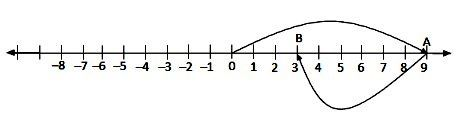(ii) On the number line, we start from 0 and move 3 steps to the left to reach point A. Now, starting from A, we move 7 steps to the right to reach point B.

B represents the integer 4.

Therefore, (-3) + 7 = 4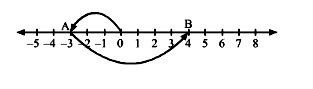(iii) On the number line, we start from 0 and move 8 steps to the right to reach point A. Now, starting from A, we move 8 steps to the left to reach point B.

B represents the integer 0.

Therefore, 8 + (-8) = 0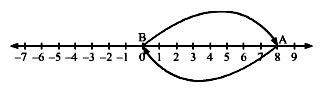(iv) On the number line, we start from 0 and move 1 step to the left to reach point A. Now, starting from A, we move 3 steps to the left to reach point B.

B represents the integer -4.

Therefore, (-1) + (-3) = -4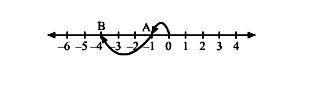(v) On the number line, we start from 0 and move 4 steps to the left to reach point A. Now, starting from A, we move 7 steps to the left to reach point B.

B represents the integer -11.

Therefore, (-4) + (-7) = -11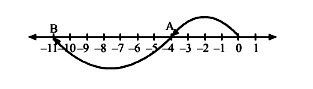(vi) On the number line, we start from 0 and move 2 steps to the left to reach point A. Now, starting from A, we move 8 steps to the left to reach point B.

B represents the integer -10.

Therefore, (-2) + (-8) = -10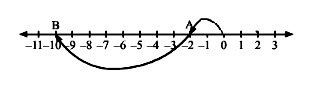(vii) On the number line, we start from 0 and move 3 steps to the right to reach point A. Now, starting from A, we move 2 steps to the left to reach point B. Again, starting from B, we move 4 steps to the left to reach point C.

C represents the integer -3.

Therefore, 3 + (-2) + (-4) = -3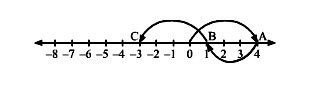(viii) On the number line, we start from 0 and move 1 step to the left to reach point A. Now, starting from A, we move 2 steps to the left to reach point B. Again, starting from B, we move 3 steps to the left to reach point C.

C represents the integer -6.

Therefore, (-1) + (-2) + (-3) = -6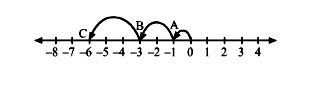(ix) On the number line, we start from 0 and move 5 steps to the right to reach point A. Now, starting from A, we move 2 steps to the left to reach point B. Again, starting from B, we move 6 steps to the left to reach point C.

C represents the integer -3.

Therefore, 5 + (-2) + (-6) = -3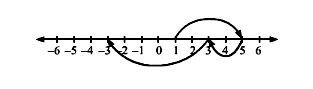Question 2:

Fill in the blanks:

(i) (-3) + (-9) = …………

(ii) (-7) + (-8) = …………

(iii) (-9) + 16 = …………

(iv) (-13) + 25 = …………

(v) 8 + (-17) = …………

(vi) 2 + (-12) = …………

Solution:

(i)

(-3) + (-9)

= -3 – 9

= -12

(ii)

(-7) + (-8)

= -7 – 8

= -15

(iii)

(-9) + 16

= -9 + 16

= 7

(iv)

(-13) + 25

= -13 + 25

= 12

(v)

8 + (-17)

= 8 – 17

= -9

(vi)

2 + (-12)

= 2 – 12

= -10

Question 3: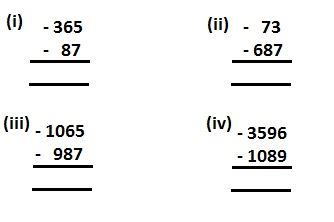Solution: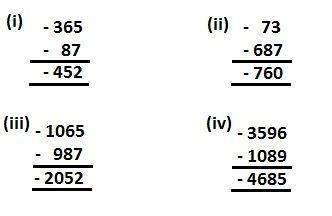Question 4: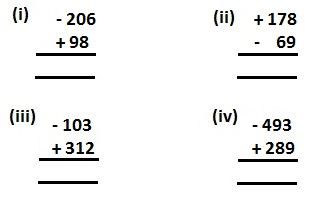Solution: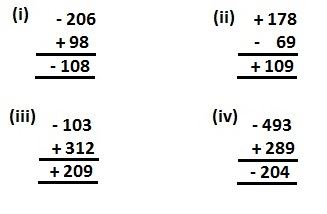Question 5:

Find the sum of

(i) 137 and -354

(ii) 1001 and -13

(iii) -3057 and 199

(iv) -36 and 1027

(v) -389 and -1032

(vi) -36 and 100

(vii) 3002 and -888

(viii) -18, +25 and -37

(ix) -312, 39 and 192

(x) -51, -203, 36 and -28

Solution:

(i)

137 + (-354)

= 137 – 354

= -217

(ii) 1001 + (-13)

= 1001 – 13

= 988

(iii) (-3057) + 199

-3057 + 199

= -2858

(iv) (-36) + 1027

= -36 + 1027

= 991

(v) (-389) + (-1032)

= -389 – 1032

= -1421

(vi) (-36) + 100

= -36 + 100

= 64

(vii) 3002 + (-888)

= 3002 – 888

= 2114

(viii) (-18) + 25 + (-37)

= -18 + 25 – 37

= -55 + 25

= -30

(ix) (-312) + 39 + 192

= -312 + 39 + 192

= -312 + 231

= -81

(x) (-51) + (-203) + 36 + (-28)

= -51 – 203 + 36 – 28

= -282 + 36

= -246

Question 6:

(i) -57

(ii) 183

(iii) 0

(iv) -1001

(v) 2054

Solution:

(i) -57 + 57 = 0

So, the additive inverse of -57 is 57.

(ii) 183 – 183 = 0

So, the additive inverse of 183 is -183.

(iii) 0 + 0 = 0

So, the additive inverse of 0 is 0.

(iv) -1001 + 1001 = 0

So, the additive inverse of -1001 is 1001.

(v) 2054 – 2054 = 0

So, the additive inverse of 2054 is -2054.

Question 7:

Write the successor of each one of the following:

(i) 201

(ii) 70

(iii) -5

(iv) -99

(v) -500

Solution:

(i) The successor of 201:

201 + 1 = 202

(ii) The successor of 70:

70 + 1 = 71

(iii) The successor of -5:

-5 + 1 = -4

(iv) The successor of -99:

-99 + 1 = -98

(v) The successor of -500:

-500 + 1 = -499

Question 8:

Write the predecessor of each one of the following:

(i) 120

(ii) 79

(iii) -8

(iv) -141

(v) -300

Solution:

(i) The predecessor of 120:

120 – 1 = 119

(ii) The predecessor of 79:

79 – 1 = 78

(iii) The predecessor of -8:

-8 – 1 = -9

(iv) The predecessor of -141:

-141 – 1 = -142

(v) The predecessor of -300:

-300 – 1 = -301

Question 9:

Simplify:

(i) (-7) + (-9) + 12 + (-16)

(ii) 37 + (-23) + (-65) + 9 + (-12)

(iii) (-145) + 79 + (-265) + (-41) + 2

(iv) 1056 + (-798) + (-38) + 44 + (-1)

Solution:

(i) (-7) + (-9) + 12 + (-16)

= 12 – (7 + 9 + 16)

= 12 – 32

= -20

(ii) 37 + (-23) + (-65) + 9 + (-12)

= 37 + 9 – (23 + 65 +12)

= 46 – 100

= -54

(iii)(-145) + 79 + (-265) + (-41) + 2

= 79 + 2 – (145 + 256 +41)

= 81 – 451

= -370

(iv) 1056 + (-798) + (-38) + 44 + (-1)

= 1056 + 44 – (798 + 38 + 1)

= 1100 – 837

= -263

Question 10:

A car travelled 60 km to the north of Patna and then 90 km to the south from there. How far from Patna was the car finally?

Solution:

Let the distance covered in the direction of north be positive and that in the direction of south be negative.

Distance travelled to the north of Patna = 60 km

Distance travelled to the south of Patna = -90 km

Total distance travelled by the car = 60 + (-90)

= -30 km

The car was 30 km south of Patna.

Question 11:

A man bought some pencils for Rs. 30 and some pens for Rs. 90. The next day, he again bought some pencils for Rs. 25. Then he sold all the pencils for Rs. 20 and the pens for Rs. 70. What was his net gain or loss?

Solution:

Total cost price = Price of pencils + Price of pens

= 30 + 90 + 25

= Rs. 145

Total amount sold = Price of pen + Price of pencils

= 20 + 70

= Rs. 90

Selling price – Cost price = 90 – 145 = -55

The negative sign implies loss.

Hence, his net loss was Rs. 55.

Question 12:

For each of the following statements write (T) for true and (F) for false:

(i) The sum of two negative integers is always a negative integer.

(ii) The sum of a negative integer and a positive integer is always a negative integer.

(iii) The sum of an integer and its negative is zero.

(iv) The sum of three different integers can never be zero.

(v) |-5| < |-3|

(vi) |8 – 5| = |8| + |-5|

Solution:

(i) True

For example: -2 + (-1) = -3

(ii) False

It can be negative or positive.

For example: -2 + 3 = 1 gives a positive integer, but -5 + 2 = -3 gives a negative integer.

(iii) True

For example: 100 + (-100) = 0

(iv) False

For example: (-5) + 2 + 3 = 0

(v) False

|-5| = 5 and |-3| = 3, 5 > 3

(vi) False

|8 – 5| = 3

|8| + |-5| = 8 + 5 = 13

Therefore, |8 – 5| $neq$ |8| + |-5|

Question 13:

Find an integer a such that

(i) a + 6 = 0

(ii) 5 + a = 0

(iii) a + (-4) = 0

(iv) -8 + a = 0

Solution:

(i) a + 6 = 0

$\Rightarrow$ a = 0 – 6

$\Rightarrow$ a = -6

(ii) 5 + a = 0

$\Rightarrow$ a = 0 – 5

$\Rightarrow$ a = -5

(iii) a + (-4) = 0

$\Rightarrow$ a – 4 = 0

$\Rightarrow$ a = 4

(iv) -8 + a = 0

$\Rightarrow$ a = 0 + 8

$\Rightarrow$ a = 8

### Key Features of RS Aggarwal Class 6 Solutions Chapter 4 – Whole Numbers Ex 4A (4.1)

• These solutions play an important role at the last minute revision.
• With the help of RS Aggarwal Maths Solution students can enhance their problem-solving skills.
• The solutions are aimed to help students while preparing for their Class 6 final exam.
• All the difficult and tricky questions are explained in a simple and easy method.

#### Practise This Question

The numbers which start from 1 are called _____.